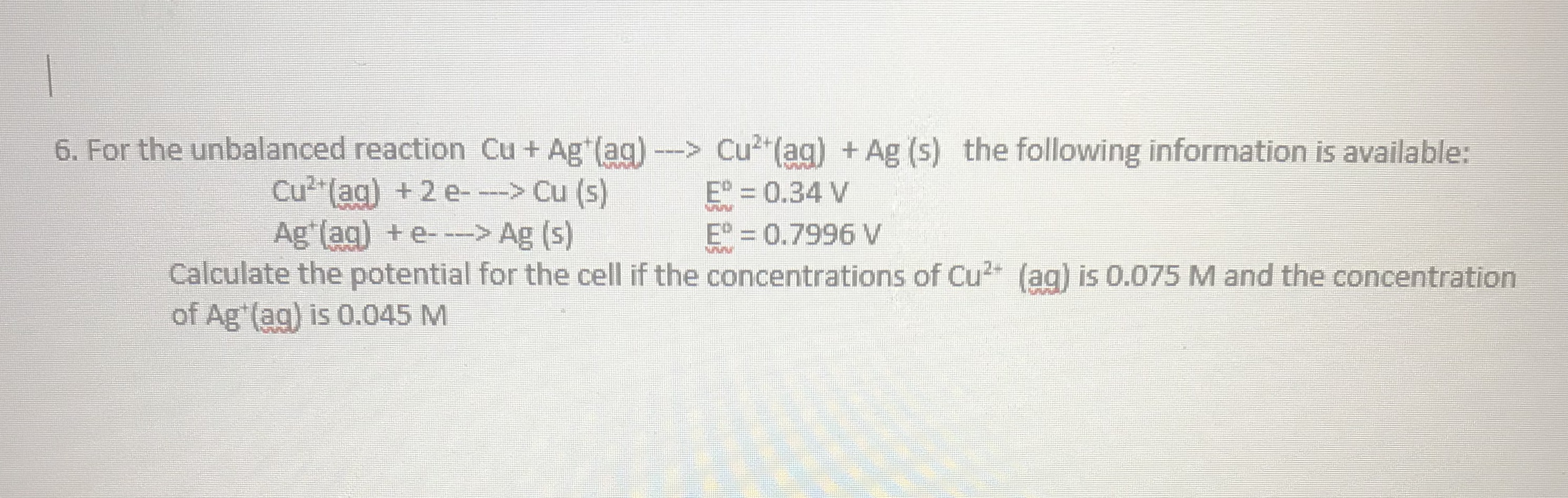# For the unbalanced reaction Cu + Ag+(aq) ---> Cu2+(aq) + Ag (s) the following information is available: ​​Cu2+(aq) + 2 e- ---> Cu (s) ​Eo = 0.34 V Ag+(aq) + e- ---> Ag (s) ​​Eo = 0.7996 V Calculate the potential for the cell if the concentrations of Cu2+ (aq) is 0.075 M and the concentration of Ag+(aq) is 0.045 M